# Lecture 15 Theory of Automata 2010 Finite Automata

• Slides: 23
Download presentationLecture 15: Theory of Automata: 2010 Finite Automata with Output National University of Computer and Emerging Sciences, FAST, Islamabad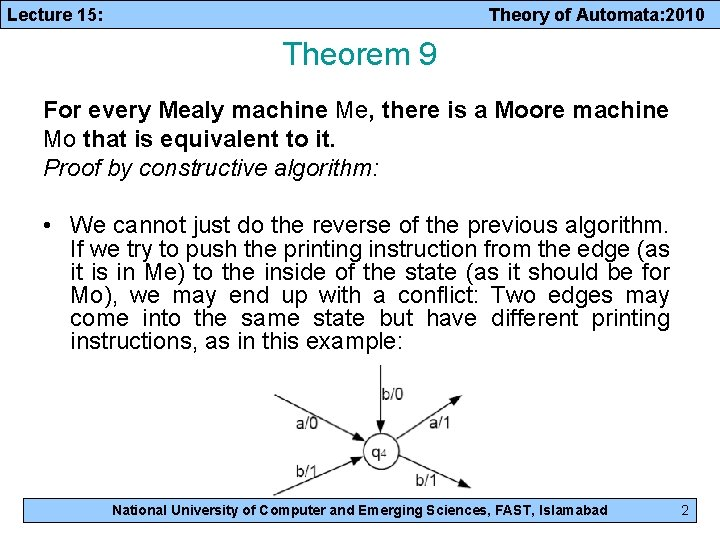Lecture 15: Theory of Automata: 2010 Theorem 9 For every Mealy machine Me, there is a Moore machine Mo that is equivalent to it. Proof by constructive algorithm: • We cannot just do the reverse of the previous algorithm. If we try to push the printing instruction from the edge (as it is in Me) to the inside of the state (as it should be for Mo), we may end up with a conflict: Two edges may come into the same state but have different printing instructions, as in this example: National University of Computer and Emerging Sciences, FAST, Islamabad 2Lecture 15: Theory of Automata: 2010 Proof by constructive algorithm (cont. ): • What we need are two copies of q 4, one that prints a 0 (labeled as q 14/0), and the other that prints a 1 (labeled as q 24/1). Hence, – The edges a/0 and b/0 will go into q 14/0. – The edge b/1 will go into q 24/1. • The arrow coming out of each of these two copies must be the same as the edges coming out of q 4 originally. National University of Computer and Emerging Sciences, FAST, Islamabad 3Lecture 15: Theory of Automata: 2010 Proof by constructive algorithm (cont. ): • If all the edges coming into a state have the same printing instruction, we simply push that printing instruction into the state. becomes National University of Computer and Emerging Sciences, FAST, Islamabad 4Lecture 15: Theory of Automata: 2010 Proof by constructive algorithm (cont. ): • An edge that was a loop in Me may becomes two edges in Mo, one that is a loop and one that is not. becomes National University of Computer and Emerging Sciences, FAST, Islamabad 5Lecture 15: Theory of Automata: 2010 Proof by constructive algorithm (cont. ): • If there is ever a state that has no incoming edges, we can assign it any printing instruction we want, even if this state is the start state. • If we have to make copies of the start state in Me, we can let any of the copies be the start state in Mo, because they all give the identical directions for proceeding to other states. • Having a choice of start states means that the conversion of Me into Mo is NOT unique. • Repeating this process for each state of Me will produce an equivalent Mo. The proof is completed. • Together, Theorems 8 and 9 allow us to say Me = Mo. National University of Computer and Emerging Sciences, FAST, Islamabad 6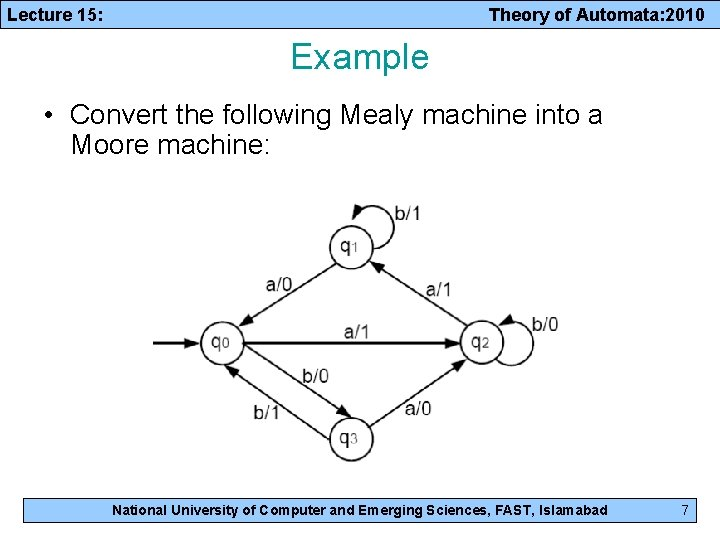Lecture 15: Theory of Automata: 2010 Example • Convert the following Mealy machine into a Moore machine: National University of Computer and Emerging Sciences, FAST, Islamabad 7Lecture 15: Theory of Automata: 2010 Example contd. • Following the algorithm, we first need two copies of q 0: National University of Computer and Emerging Sciences, FAST, Islamabad 8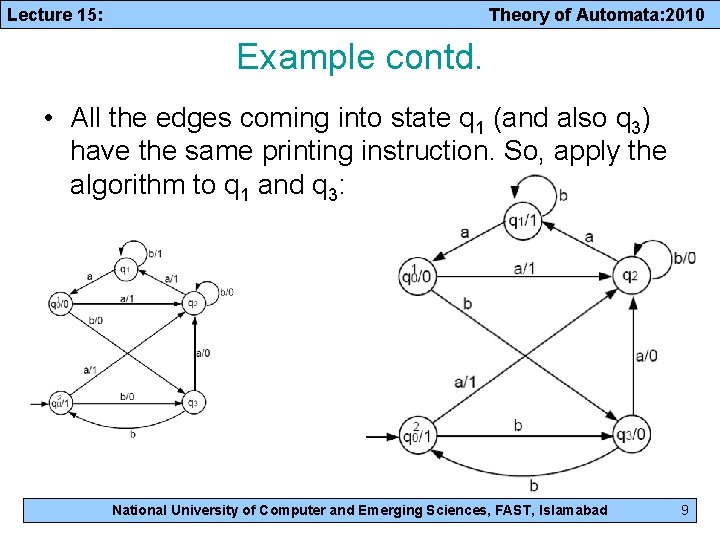Lecture 15: Theory of Automata: 2010 Example contd. • All the edges coming into state q 1 (and also q 3) have the same printing instruction. So, apply the algorithm to q 1 and q 3: National University of Computer and Emerging Sciences, FAST, Islamabad 9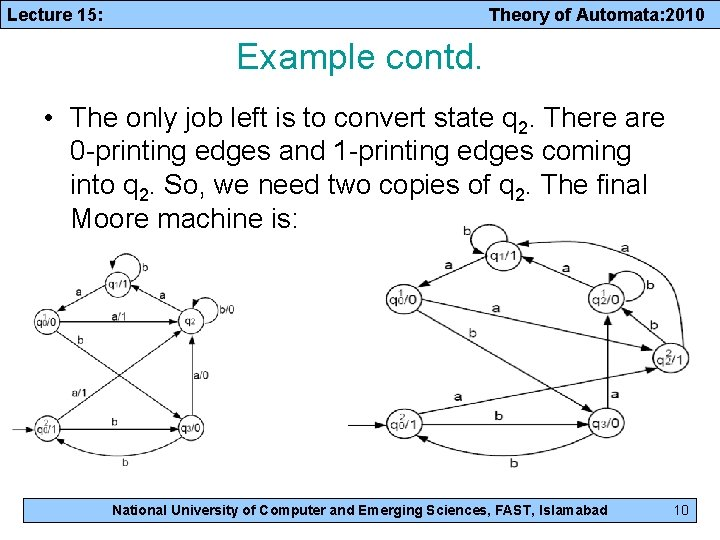Lecture 15: Theory of Automata: 2010 Example contd. • The only job left is to convert state q 2. There are 0 -printing edges and 1 -printing edges coming into q 2. So, we need two copies of q 2. The final Moore machine is: National University of Computer and Emerging Sciences, FAST, Islamabad 10Lecture 15: Theory of Automata: 2010 • Closure Properties • Complementation • Intersection National University of Computer and Emerging Sciences, FAST, Islamabad 11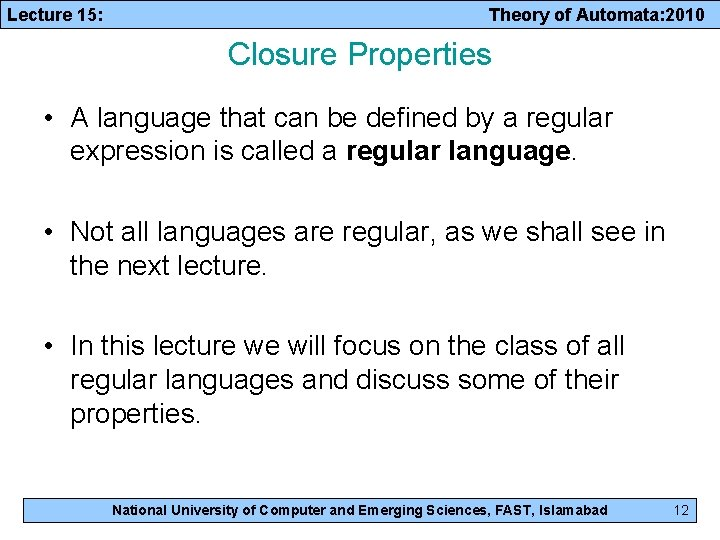Lecture 15: Theory of Automata: 2010 Closure Properties • A language that can be defined by a regular expression is called a regular language. • Not all languages are regular, as we shall see in the next lecture. • In this lecture we will focus on the class of all regular languages and discuss some of their properties. National University of Computer and Emerging Sciences, FAST, Islamabad 12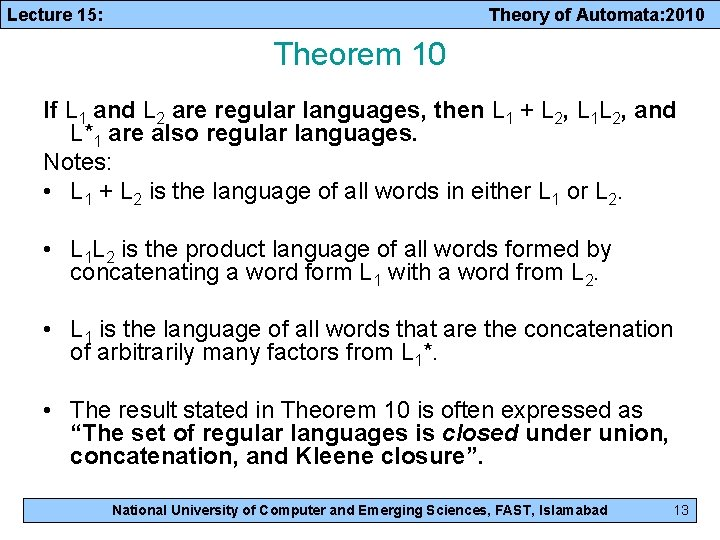Lecture 15: Theory of Automata: 2010 Theorem 10 If L 1 and L 2 are regular languages, then L 1 + L 2, L 1 L 2, and L*1 are also regular languages. Notes: • L 1 + L 2 is the language of all words in either L 1 or L 2. • L 1 L 2 is the product language of all words formed by concatenating a word form L 1 with a word from L 2. • L 1 is the language of all words that are the concatenation of arbitrarily many factors from L 1*. • The result stated in Theorem 10 is often expressed as “The set of regular languages is closed under union, concatenation, and Kleene closure”. National University of Computer and Emerging Sciences, FAST, Islamabad 13Lecture 15: Theory of Automata: 2010 Proof by Machines • Because L 1 and L 2 are regular languages, there must be TGs that accept them (by Kleene’s theorem). • Let TG 1 accepts L 1 and TG 2 accepts L 2. • Assume that TG 1 and TG 2 each have a unique start state and a unique separate final state. If this is not the case originally, then we can modify the TGs so that this becomes true as in Kleene’s theorem, Part 2 of the proof (page 93). National University of Computer and Emerging Sciences, FAST, Islamabad 14Lecture 15: Theory of Automata: 2010 Proof contd. • Then the TG described below accepts the language L 1 + L 2. • By Kleene’s theorem, since L 1 + L 2 is defined by this TG, it is also defined by a regular expression and hence is a regular language. National University of Computer and Emerging Sciences, FAST, Islamabad 15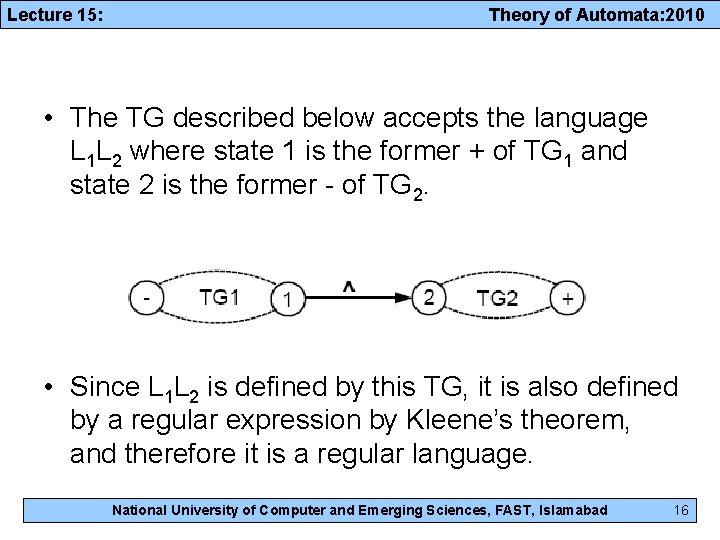Lecture 15: Theory of Automata: 2010 • The TG described below accepts the language L 1 L 2 where state 1 is the former + of TG 1 and state 2 is the former - of TG 2. • Since L 1 L 2 is defined by this TG, it is also defined by a regular expression by Kleene’s theorem, and therefore it is a regular language. National University of Computer and Emerging Sciences, FAST, Islamabad 16Lecture 15: Theory of Automata: 2010 • The TG described below accepts the language L 1*. • We begin at the - state and trace a path to the + state of TG 1. At this point, we cold stop and accept the string or jump back, at no cost, to the - state and run another segment of the input string. National University of Computer and Emerging Sciences, FAST, Islamabad 17Lecture 15: Theory of Automata: 2010 Complements. Intersections National University of Computer and Emerging Sciences, FAST, IslamabadLecture 15: Theory of Automata: 2010 Complements Definition: • If L is a language over the alphabet , we define its complement L` to be the language of all strings of letters from that are not words in L. Example: – Let L be the language over the alphabet ∑ = {a; b} of all words that have a double a in them. – Then, L` is the language of all words that do not have a double a in them. • Note that the complement of L` is L. That is (L`)` = L National University of Computer and Emerging Sciences, FAST, Islamabad 19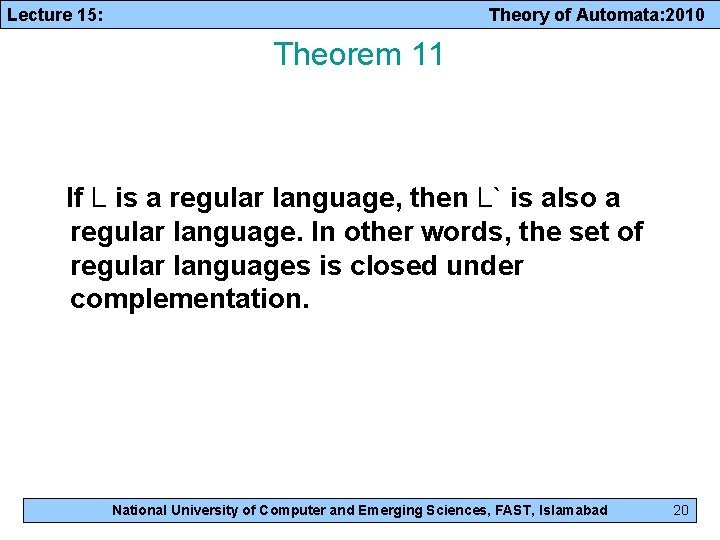Lecture 15: Theory of Automata: 2010 Theorem 11 If L is a regular language, then L` is also a regular language. In other words, the set of regular languages is closed under complementation. National University of Computer and Emerging Sciences, FAST, Islamabad 20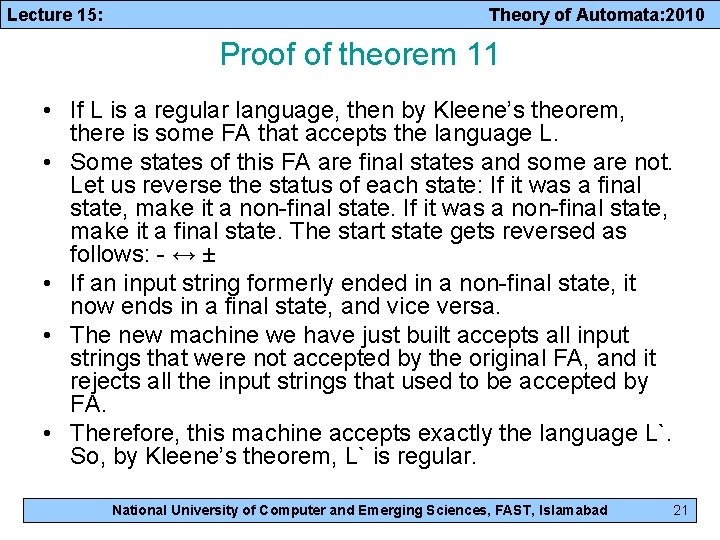Lecture 15: Theory of Automata: 2010 Proof of theorem 11 • If L is a regular language, then by Kleene’s theorem, there is some FA that accepts the language L. • Some states of this FA are final states and some are not. Let us reverse the status of each state: If it was a final state, make it a non-final state. If it was a non-final state, make it a final state. The start state gets reversed as follows: - ↔ ± • If an input string formerly ended in a non-final state, it now ends in a final state, and vice versa. • The new machine we have just built accepts all input strings that were not accepted by the original FA, and it rejects all the input strings that used to be accepted by FA. • Therefore, this machine accepts exactly the language L`. So, by Kleene’s theorem, L` is regular. National University of Computer and Emerging Sciences, FAST, Islamabad 21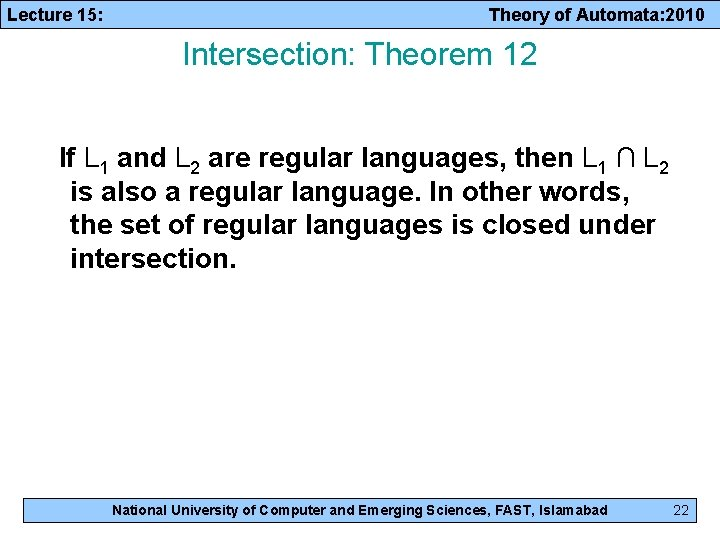Lecture 15: Theory of Automata: 2010 Intersection: Theorem 12 If L 1 and L 2 are regular languages, then L 1 ∩ L 2 is also a regular language. In other words, the set of regular languages is closed under intersection. National University of Computer and Emerging Sciences, FAST, Islamabad 22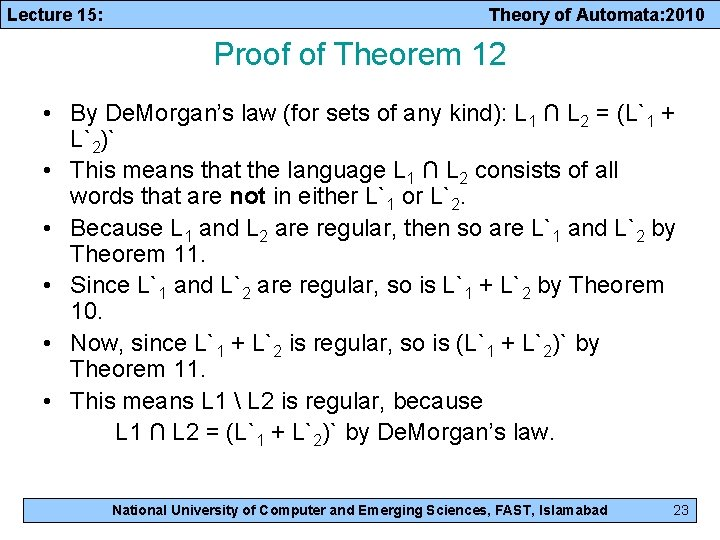Lecture 15: Theory of Automata: 2010 Proof of Theorem 12 • By De. Morgan’s law (for sets of any kind): L 1 ∩ L 2 = (L`1 + L`2)` • This means that the language L 1 ∩ L 2 consists of all words that are not in either L`1 or L`2. • Because L 1 and L 2 are regular, then so are L`1 and L`2 by Theorem 11. • Since L`1 and L`2 are regular, so is L`1 + L`2 by Theorem 10. • Now, since L`1 + L`2 is regular, so is (L`1 + L`2)` by Theorem 11. • This means L 1 L 2 is regular, because L 1 ∩ L 2 = (L`1 + L`2)` by De. Morgan’s law. National University of Computer and Emerging Sciences, FAST, Islamabad 23